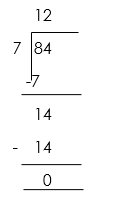# DIVISION OF WHOLE NUMBERS

##### HELLO, WELCOME BACK TO CLASS

Division is breaking a number up into an equal number of parts. Division is splitting into equal parts or groups. It is the result of “fair sharing”.

###### Signs for Division

There are a number of signs that people may use to indicate division. The most common one is ÷, but the backslash / is also used. Sometimes people will write one number on top of another with a line between them. This is also called a fraction.

###### Dividend, Divisor and Quotient

Each part of a division equation has a name. The three main names are the dividend, the divisor, and the quotient.

• Dividend – The dividend is the number you are dividing up
• Divisor – The divisor is the number you are dividing by
• Quotient – The quotient is the answer

### Dividend ÷ Divisor = QuotientWord problem

7 girls are to share 84 oranges. How many will each get?Quiz

1. Divide 393 by 3
2. Divide 460 by 4
3. 4 students are to share 84 berries. How many will each get?

How Can We Make ClassNotesNG Better? - CLICK to Tell Us Now!

1.